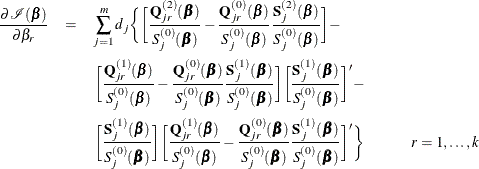# The PHREG Procedure

### Firth’s Modification for Maximum Likelihood Estimation

In fitting a Cox model, the phenomenon of monotone likelihood is observed if the likelihood converges to a finite value while at least one parameter diverges (Heinze and Schemper, 2001).

Let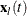denote the vector explanatory variables for the lth individual at time t. Let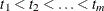denote the k distinct, ordered event times. Letdenote the multiplicity of failures at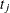; that is,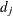is the size of the set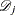of individuals that fail at. Let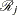denote the risk set just before. Let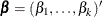be the vector of regression parameters. The Breslow log partial likelihood is given by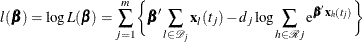Denote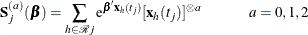Then the score function is given by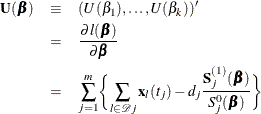and the Fisher information matrix is given by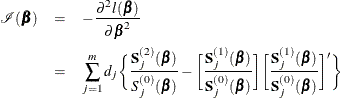Heinze (1999); Heinze and Schemper (2001) applied the idea of Firth (1993) by maximizing the penalized partial likelihood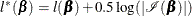The score function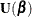is replaced by the modified score function by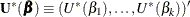, where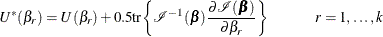The Firth estimate is obtained iteratively as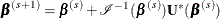The covariance matrix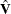is computed as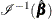, where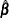is the maximum penalized partial likelihood estimate.

#### Explicit formulae for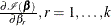Denote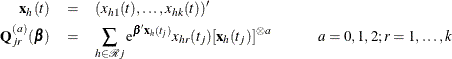Then